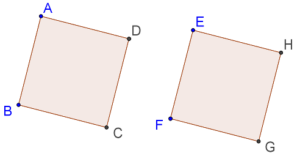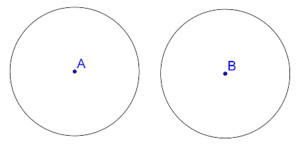# What is Congruence

Go back to  'Triangles-Quadrilaterals'

Two geometrical figures are said to be congruent if they are identical in every respects. For example, two squares of the same side-length are congruent, as shown below:Similarly, two circles with the same radius are congruent:If two geometrical figures are congruent, they can be exactly superimposed upon each other.

If a figure A is congruent to a figure B, we will write this fact as follows: A ≡ B. Notice the following obvious facts about congruence:

1. If A ≡ B, then B ≡ A. This simply means that saying that A is congruent to B is the same as saying that B is congruent to A.

2. If A ≡ B and B ≡ C, then A ≡ C. This should once again be easy to understand. If A is congruent to B, it can be superimposed exactly on B. Similarly, B can be superimposed exactly on C. Thus, A can be superimposed exactly on C, and hence A ≡ C.